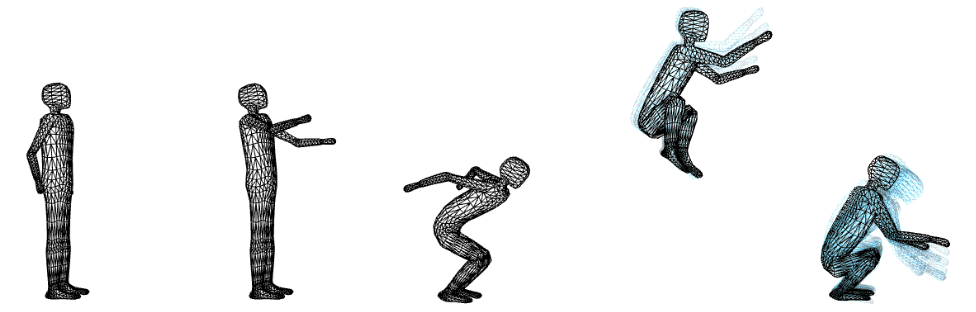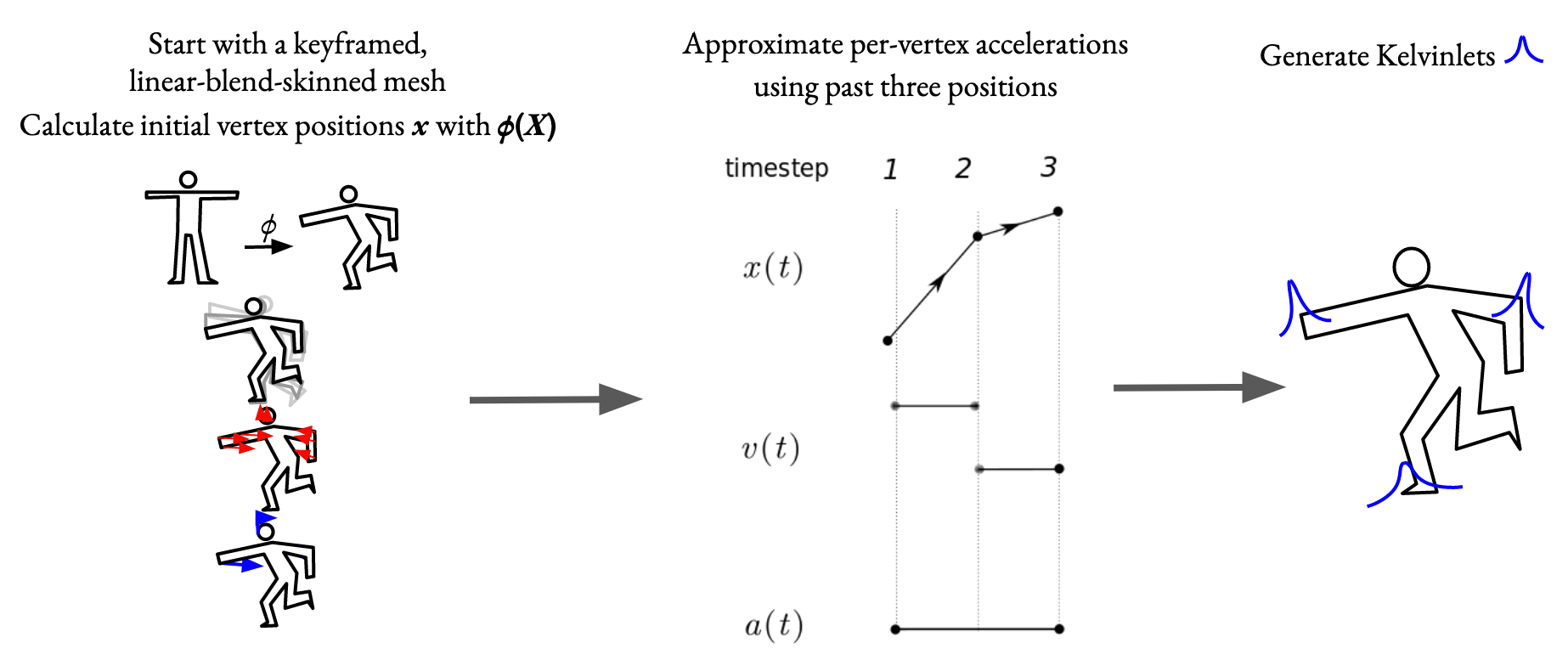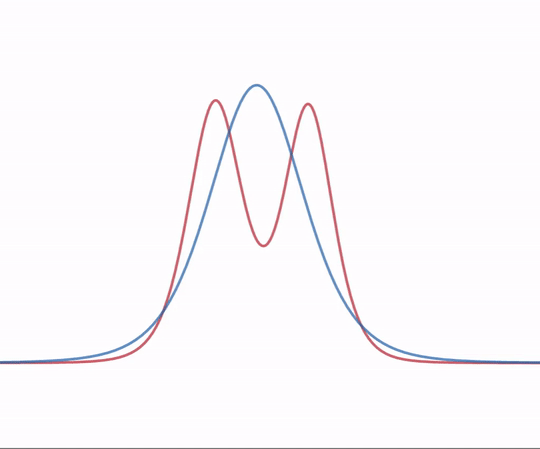# Automatic Secondary Motion with Dynamic Kelvinlets

Written in collaboration with Jasmeet Singh.
December 1, 2019

Artists commonly use physics simulations to add extra detail and realism to animations. However, most physics simulations run slowly on standard hardware, potentially require a volumetric mesh, and are often hard to control. We set out to take as input simple skeletal animation and add squash and stretch, overshoot, and jiggling automatically in real time.

Dynamic Kelvinlets by James et al. offer a potential solution: they model elastic deformations and are fast to compute. They compute the response to regularized forces, which are forces spread out over some area instead of being concentrated at a single point. They operate on an infinite continuum, which means that they don't care about the shape of your mesh; instead, they treat your mesh vertices like they are floating in an infinite block of Jello, and simply sends waves through the Jello. Pixar lets artists use these as tools in their animation toolbox by manually placing them in scenes.

## Kelvinlet generation scheme

Our system automatically places these Dynamic Kelvinlets into the scene in real time based on the changes each frame. First, we apply the artist-made skeletal animation to come up with updated vertex positions. Then, we approximate velocity and acceleration of each vertex by comparing with the previous two frames. Using these accelerations, we place a Kelvinlet at that location. We compute an approximate mass at each vertex and give the Kelvinlet enough force to create the observed acceleration.Creation of new Kelvinlets each frame based on vertex positions, velocities, and accelerations

To keep the simulation real-time, we need to limit the number of active Kelvinlets. To do so, we iteratively merge nearby Kelvinlets into a single Kelvinlet that approximating their sum. We pick Kelvinlets to merge whose force vectors are roughly aligned, and whose radii are touching (where the radius is defined as the distance at which the force reaches 10% of the force at its center.) We pick the force and surface area spread of merged Kelvinlets such that

• the volume of the replacement Kelvinlet is the same as the volume of the sum of the originals, and
• as the force of either original goes down to 0, the combined Kelvinlet converges to the other nonzero Kelvinlet.The merged Kelvinlet (blue) and the sum of the two input Kelvinlets (red)